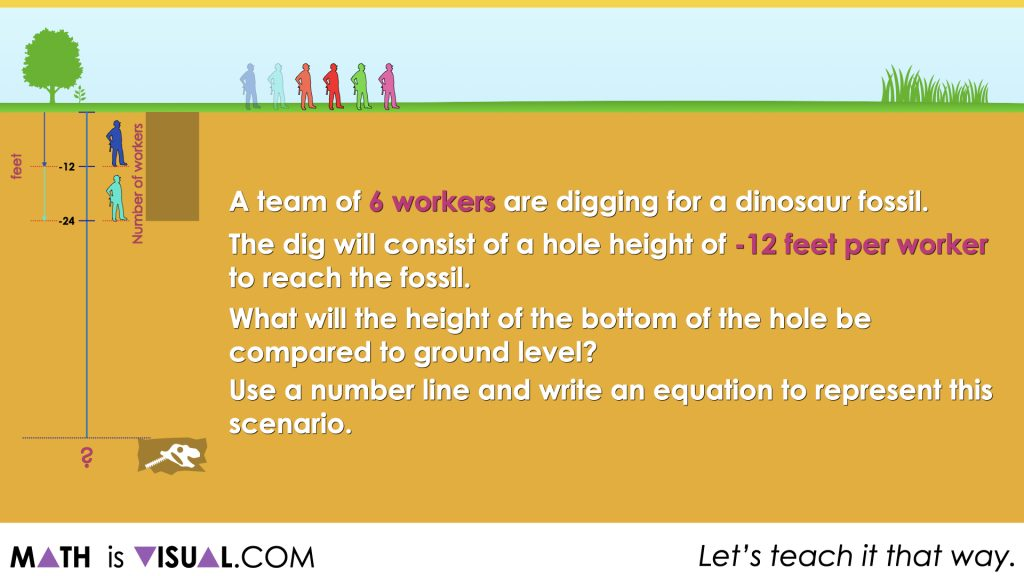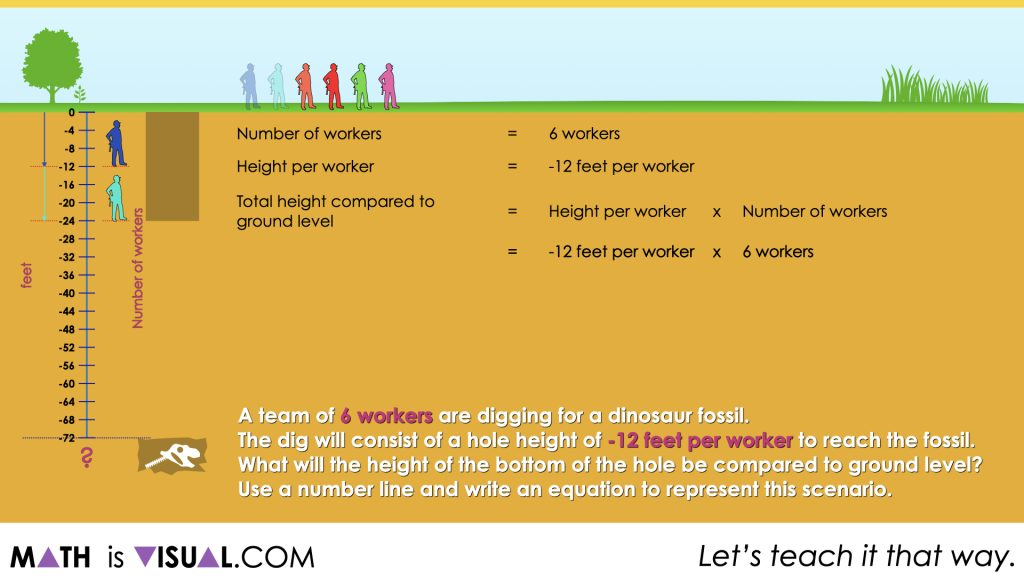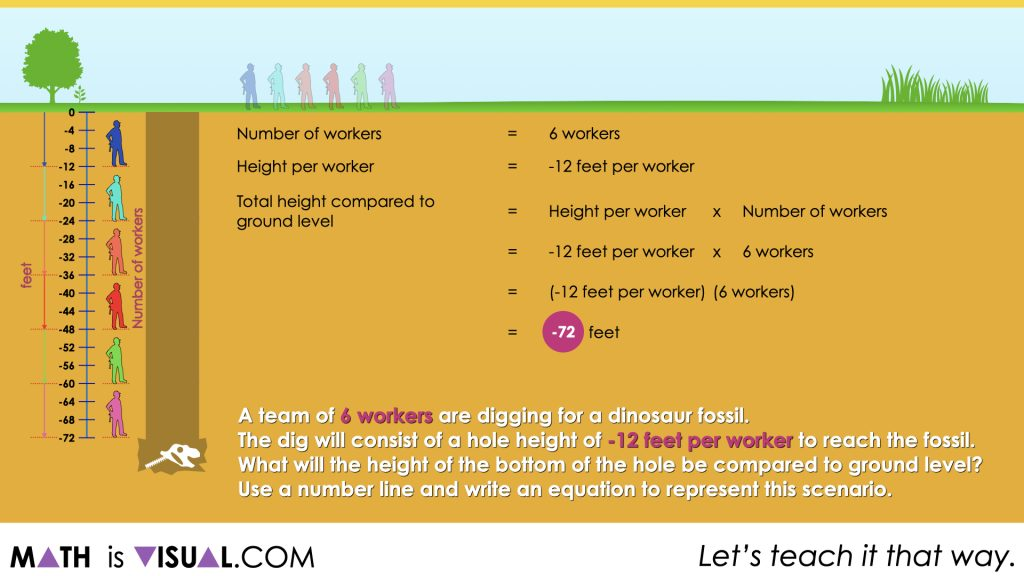# Multiplying and Dividing Negative Numbers

Build a deeper understanding of the behaviours of multiplying and dividing positive and negative integers through a visual number talk.

## In This Set of Visual Number Talk Prompts…

Through this math talk and purposeful practice, students are encouraged to model the behaviour of multiplying and dividing positive and negative integers. All division scenarios today will be partitive.

## Intentionality…

The purpose of the Day 4 activities is to reinforce key concepts from Day 3. Students will continue to explore examples of partitive division involving a negative and positive integer. Students will engage in a math talk that will serve to reinforce the relationship between multiplication and division. They will independently complete purposeful practice to develop a deeper understanding of the following big ideas.

• Partitive division is when the total quota is known (the dividend), and the number of parts or groups (the divisor) is known;
• Partitive division reveals a rate;
• In partitive division, the dividend and the divisor often have different units;
• A negative number divided by a positive number has a quotient that is negative.

## Visual Math Talk Prompt #1

Students will be prompted with:

A team of 6 workers are digging for a dinosaur fossil.

The dig will require the hole height compared to ground level to change by -12 feet per worker to reach the fossil.

What will the height at the bottom of the hole be compared to ground level?

Use a number line and write an equation to represent this scenario.The goal of this particular prompt is to get students thinking about the result when you multiply a positive number by a negative. In this case, the number of groups or iterations is positive and the rate is negative. Therefore, we are iterating a negative rate and the result should be a negative number. As workers repeatedly dig 12 feet further into the ground, the height at the bottom of the hole is more negative than it was the worker before.

6 people x -12 feet per person = -72 feet

And

-72 feet ÷ 6 people = -12 feet per personThe next image makes it clear that the length (or height) of the hole is 72 feet into the ground or -72 feet relative to ground level.## Want to Explore These Concepts & Skills Further?

Explore additional number talk prompts in Day 4 of the Just Keep Digging problem based math unit that you can dive into now.

Why not start from the beginning of this contextual 5-day unit of real world lessons from the Make Math Moments Problem Based Units page.

Did you use this in your classroom or at home? How’d it go? Post in the comments!

Math IS Visual. Let’s teach it that way.